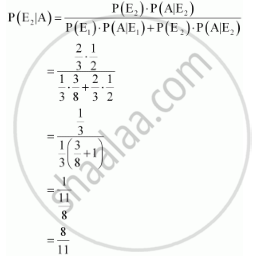Share

# Suppose a Girl Throws a Die. If She Gets a 5 Or 6, She Tosses a Coin Three Times and Notes the Number of Heads. If She Gets 1, 2, 3 Or 4, She Tosses a Coin Once and Notes Whether a Head Or Tail is Obtained. If She Obtained Exactly One Head, What is the Probability that She Threw 1, 2, 3 Or 4 with the Die? - CBSE (Science) Class 12 - Mathematics

#### Question

Suppose a girl throws a die. If she gets a 5 or 6, she tosses a coin three times and notes the number of heads. If she gets 1, 2, 3 or 4, she tosses a coin once and notes whether a head or tail is obtained. If she obtained exactly one head, what is the probability that she threw 1, 2, 3 or 4 with the die?

#### Solution

Let E1 be the event that the outcome on the die is 5 or 6 and E2 be the event that the outcome on the die is 1, 2, 3, or 4.

:. P(E_1) = 2/6 = 1/3 and P(E_2) = 4/6  = 2/3

Let A be the event of getting exactly one head.

P (A|E1) = Probability of getting exactly one head by tossing the coin three times if she gets 5 or 6 = 3/8

P (A|E2) = Probability of getting exactly one head in a single throw of coin if she gets 1, 2, 3, or 4  = 1/2

The probability that the girl threw 1, 2, 3, or 4 with the die, if she obtained exactly one head, is given by P (E2|A).

By using Bayes’ theorem, we obtainIs there an error in this question or solution?

#### APPEARS IN

Solution Suppose a Girl Throws a Die. If She Gets a 5 Or 6, She Tosses a Coin Three Times and Notes the Number of Heads. If She Gets 1, 2, 3 Or 4, She Tosses a Coin Once and Notes Whether a Head Or Tail is Obtained. If She Obtained Exactly One Head, What is the Probability that She Threw 1, 2, 3 Or 4 with the Die? Concept: Baye'S Theorem.
S